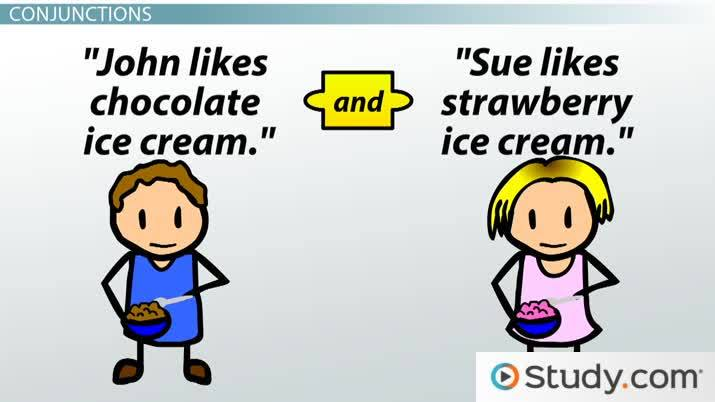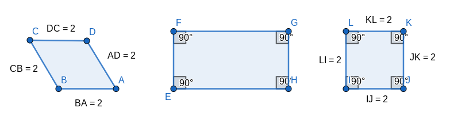# Conjunction and Disjunction in Math: Distinctions and Properties

Emma May, Yuanxin (Amy) Yang Alcocer
• Author
Emma May

Emma May is a mathematician with a bachelor's degree in mathematics from Vassar College. She has tutored subjects such as calculus, linear algebra, and multivariable calculus for over three years. She also conducted mathematics research in topics such as combinatorics and dynamics for over four years.

• Instructor
Yuanxin (Amy) Yang Alcocer

Amy has a master's degree in secondary education and has been teaching math for over 9 years. Amy has worked with students at all levels from those with special needs to those that are gifted.

A conjunction is an 'and' statement in mathematics. Disjunction is an 'or' statement in mathematics. They share some similarities and differences. Updated: 12/25/2021

Show

## Conjunction in Math

The study of logic statements, holding values true or false, is called Boolean algebra. There are two types of connective logic that are important to learn. The first is conjunction. A conjunction statement is a statement involving an and. For example, a square has four equal sides and four equal measure angles. If a four-sided polygon does not have four equal sides and four equal measure angles, then it is not a square. Both sides of the and expression must have either the value of being true or false. To write an and statement using mathematical notation, use the {eq}\wedge {/eq} symbol. If p and q are statements with value either true or false, then the conjunction of p with q is written {eq}p \wedge q {/eq}. It is important to note that the conjunction of two statements is another statement, which also has the value: true or false. Consider another example. The conjunction of the statement "The sky is blue" with "the grass is green" is "The sky is blue, and the grass is green."An error occurred trying to load this video.

Try refreshing the page, or contact customer support.

Coming up next: Conditional Statements in Math

### You're on a roll. Keep up the good work!

Replay
Your next lesson will play in 10 seconds
• 0:05 Statements
• 0:36 Connectors
• 0:58 Conjunctions
• 1:59 Disjunctions
• 2:53 Lesson Summary
Save Save

Want to watch this again later?

Timeline
Autoplay
Autoplay
Speed Speed

## Conjunction Statement

The conjunction statement of two statements p and q is {eq}p\wedge q {/eq}, as briefed earlier. This means "p and q." The conjunction statement itself is either true or false. It is only true when both p and q are true. If one of either p or q is false, then the statement is false. It makes sense that conjunction is known as an 'and' statement because p and q must be true for the statement to be true. For example, suppose that there is a four-sided polygon S that is a square. Then it is true that the S has four equal sides, and it is true S has four equal angles. Hence, it is true that S has four equal sides length AND four equal measure angles.If either a four-sided polygon has only four equal angles or four equal length sides, it is possible that they are not squares. It is only definitively true that the polygon is a square if it has four equal sides and four equal measure angles.

## Disjunction statement

The other important form of connective logic is disjunction. A conjunction is a statement involving an or. For two statements p and q, it is written in mathematical notation as {eq}p \vee q {/eq}. Again, both p and q have to be either true or false statements. Again, the disjunction of two statements is another statement. For example, suppose that {eq}x^2 -4x + 3 = 0 {/eq}. Then, either x = 3 or x = 1. Consider another example of a disjunction. The disjunction of the statements "The stop light is green" and "the stop light is red" is "The stop light is red or the stop light is green."

## Disjunction Statement

A disjunction statement of two statements p and q is written as {eq}p \vee q {/eq}. This means p or q. Again, p and q both need to be statements with a true or false value. To evaluate a disjunction statement if it is true, either one or both of the statements ought to be true. In other words, either p or q is true. Consider the example again, supposing that {eq}x^2 - 4x + 3 = 0 {/eq}. It is true that either x = 3 or x = 1. To check this, {eq}3^2 -4*3 + 3 = 9 - 12 + 3 = -3 + 3 = 0 {/eq}, and {eq}1^2 - 4*1 +3 = 1 - 4 + 3= -3 + 3 = 0 {/eq}. Thus, statement x = 3 or x = 1 is true, because both x = 3 and x = 1 satisfy the quadratic equation.

To unlock this lesson you must be a Study.com Member.

#### What is a conjunction statement?

A conjunction statement is a statement using 'and'. The conjunction is only valid when both of the statements in the conjunction are true.

#### What is a disjunction statement?

A disjunction statement is a statement using 'or'. The disjunction statement is true if one or both parts of the disjunction are true.

#### What is an example of a conjunction statement in math?

Suppose there is a polygon, which is a square. Then, it is true that "the polygon has four equal length sides and four equal angles."

#### What is an example of a disjunction statement in math?

A simple example of a disjunction is the statement x < 0 or x > 1. It means x is less than 0 or x is greater than 1.

#### How to write a conjunction statement?

To create a conjunction statement, join two statements using 'and'. Consider these two statements: "the cow goes moo" and "the dog goes bark." The conjunction of these two statements is "the cow goes moo, and the dog goes bark."

### Register to view this lesson

Are you a student or a teacher?